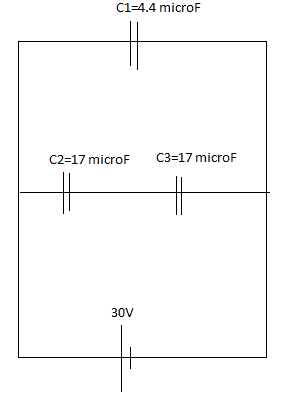# Pd accross capacitance

jake jungle

## Homework StatementFind the pd accros C2

Q=CV

## The Attempt at a Solution

i find C23 = (C2C3)/ (C2 + C3)
then find Q23 = V x C23
Q23 = Q2 = C2V2 => V2

i used this and got V2 = 15V , however the answer is 20V , and i even didnt use the data of C1 . am i wrong somewhere ?